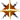### The following questions relate to the Transistor Ckt Schematic available here:

####Transistor Ckts Schematic (pdf) - 8 Selections

##### General Questions:
• Identify by the name or purpose of Q1 in Figure #1
• Identify by the name of purpose of the specific circuits Figure #3, #4, #5, #7, and #8
• In the circuits shown as Figures #2, #6, and #7, Q1 and Q2 form what is commonly called a ___________ circuit.
• In the circuit shown as Figure #5, Q1 and Q2 have what things in common with Q2 and Q3 of Figure #8? _____________________________________________
• What circuit in this group of circuits is commonly used for analog purposes? ___________
• The one circuit which is known for it's hysteresis switching effect is the circuit shown as Figure # ___.
##### Questions about the circuit shown as Figure #1:

Note: Where a (.... / ... ) is shown, it represents a choice of those items within the parenthesis.

• Q1 represents a (low/high) dynamic resistance to point "B".
• Point "E" should be (left as-is / -V / +V) for Q2 to work correctly.
• Q1 represents a (constant-current / constant-voltage) source, and Q2 represents a (constant-current / constant-voltage) source.
##### Questions about the circuit shown as Figure #2:
• Solve for all currents, IR drops, and potentials, using the values as given.
• What would be the minimum value of R1 that could be used before Q2 saturates? _______________
• Q1 and Q2 form what is commonly called a _________ circuit, and the purpose of this circuit is to function as a _________   ______________.
##### Questions about the circuit shown as Figure #7:
• Solve for all currents, IR drops, and potentials, using the values as given.
##### Questions about the circuit shown as Figure #6:
• Solve for all currents, IR drops, and potentials, using the values as given.
• What value of input current would cause Q2 to saturate? ( ______ micro-amps)
• What value of input current would cause Q1 to saturate? ( ______ micro-amps)Return to "Main Page"Return to "Study Guides"Return to "Transistors"

Tran_Ckt_ID.html - SfE-DCS, ddf - 12/28/2001